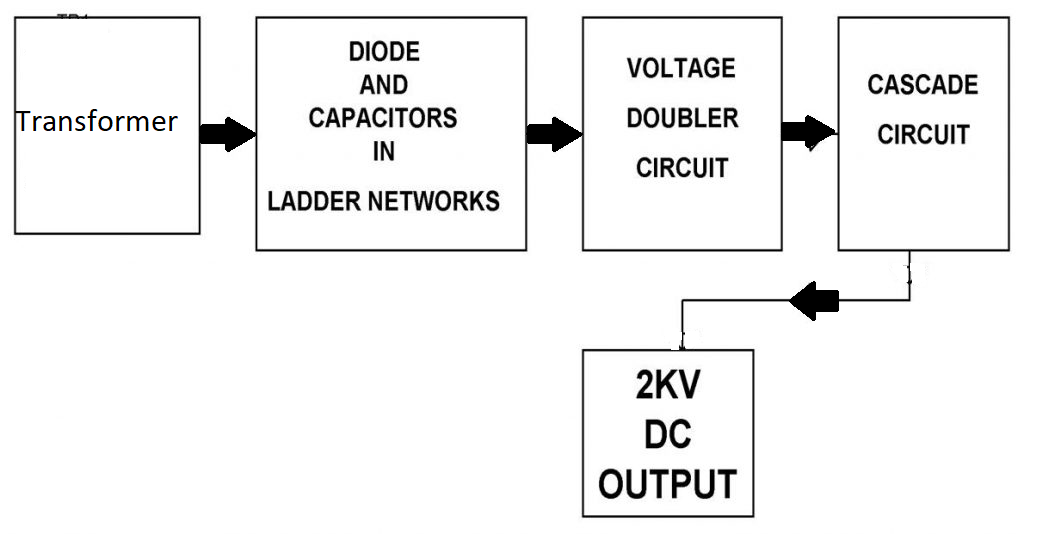# Ac to High Voltage DC Using Voltage Multiplier CircuitOur project aims to develop a system that develops a high voltage DC upto 2KVolt using a 230V Ac input. The circuit uses capacitors along with diodes in a ladder based network as a voltage multiplier mechanism.
Here we use a step by step transformer in order to step up the voltage. The secondary of the transformer output increases the voltage thus decreasing the current. Another way to step up the voltage is by making use of a voltage multiplier ckt. We mostly use voltage multipliers in order to provide high voltage in cases where low current is needed. Our system defines the mechanism to develop DC high voltage (upto 10 KiloV) from an AC single phase supply. In order to adhere to safety precautions our system restricts the multiplication factor to eight, in order to keep the output within 2KVolt. We use this concept in various electronic components including, oscilloscope, TV picture tubes, Washing machines as well as other industrial devices. The systems consist of voltage multipliers whose duty is to keep on multiplying the voltage at every single stage. So the output we get from an 8 step multiplier is multiplies upto 2Kv. Since it is not possible measuring output using basic multimeters, we here use 10:1 potential divider where a 200 V reading is considered as 2Kv. The low impedence measurement here means the output is approximately seven times the input AC voltage.• Hardware Specifications
• Multimeter
• Capacitor
• Bulb holder
• Resistors
• Diode
• Cables and Connectors
• PCB

Block Diagram